0

# python实现一个学生管理系统

``````                         2、熟悉对txt文本文件的增删改查

3、熟悉对字典、列表、字符串的处理
``````

1. 添加学生及成绩信息
2. 将学生信息保存到文件中
3. 修改和删除学生信息
4. 查询学生信息
5. 根据学生成绩进行排序
6. 统计学生的总分

## 二、系统功能结构——学生信息系统的7大模块

1. 录入学生信息
2. 查找学生信息
3. 删除学生信息
4. 修改学生信息
5. 学生成绩排名模块
6. 统计学生总人数模块
7. 显示全部学生信息模块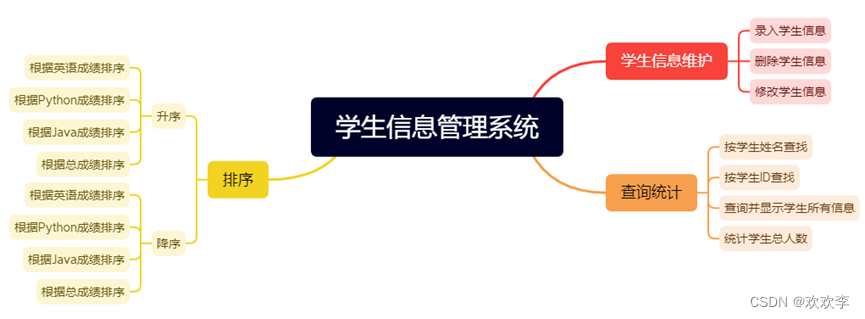## 三、系统业务流程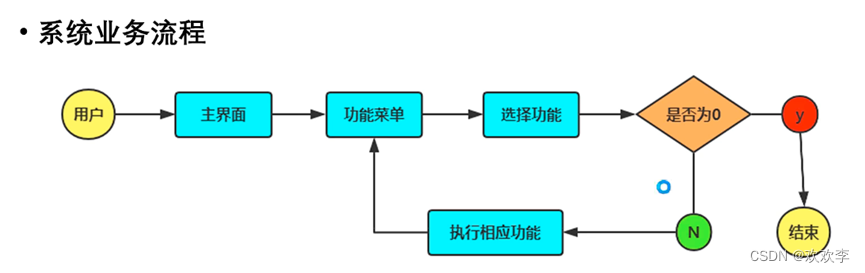## 四、开发环境

``````     操作系统：win10

Python解释器版本：python3.10

开发工具：Pycharm

Python内置模块：os,re
``````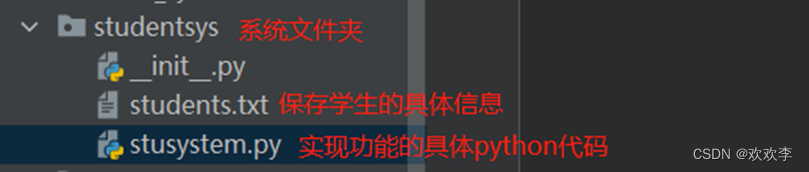## 五、主函数的业务流程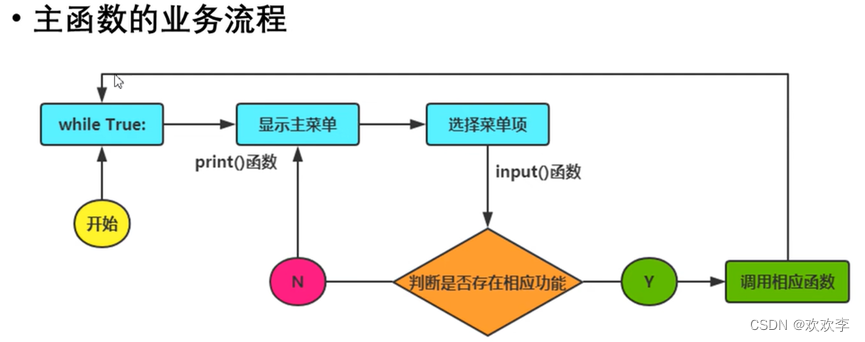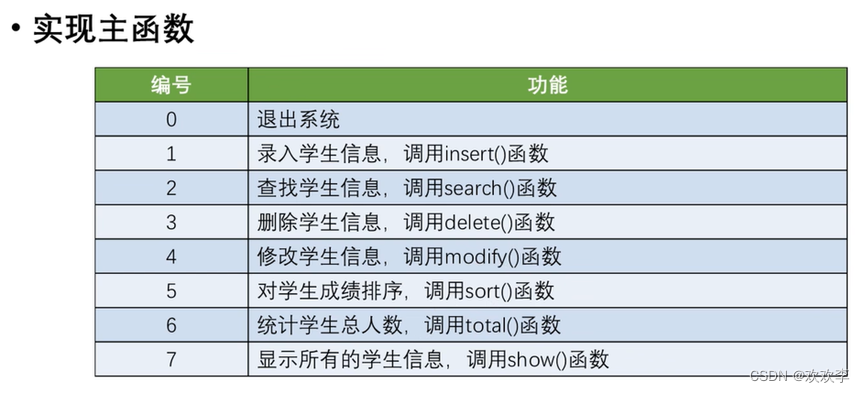主函数主要是以上这些，在开发过程中，因为涉及到重复性的操作，我又写了一些函数封装了

## 六、实现代码如下（只是实现了主流程，很多细节上的东西还需要调整）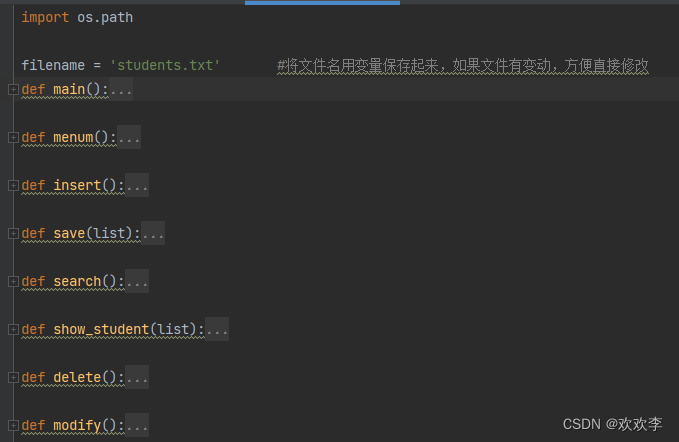``````import os.path

filename = 'students.txt'       #将文件名用变量保存起来，如果文件有变动，方便直接修改
def main():     #系统运行过程中可循环选择使用系统哪个功能
while True:
choice = int(input('请选择:'))
if choice in [0,1,2,3,4,5,6,7]:
if choice == 0:
print('感谢您的使用！！！')
break
else:
continue
elif choice == 1:
insert()    #录入学生信息
elif choice == 2:
search()    #查询学生信息
elif choice == 3:
delete()    #删除学生信息
elif choice == 4:
modify()    #修改学生信息
elif choice == 5:
sort()    #对学生成绩排序
elif choice == 6:
total()    #统计学生总人数
elif choice == 7:
show()      #显示所有学生信息
else:
print('输入有误，请重新输入')

print('===========================学生信息管理系统=========================')
print('-------------------------------功能菜单--------------------------------')
print('\t\t\t\t\t\t\t1、录入学生信息')
print('\t\t\t\t\t\t\t2、查找学生信息')
print('\t\t\t\t\t\t\t3、删除学生信息')
print('\t\t\t\t\t\t\t4、修改学生信息')
print('\t\t\t\t\t\t\t5、排序')
print('\t\t\t\t\t\t\t6、统计学生总人数')
print('\t\t\t\t\t\t\t7、显示所有学生信息')
print('\t\t\t\t\t\t\t0、退出')
print('-------------------------------------------------------------------------')

def insert():
# 调用get_student() 函数，读取当前存储学生信息的txt文本中学生的信息，并将学生信息转换为字符串，具体可看get_student() 函数
#因为多次地方会涉及到读取，所以我就直接将这个方法封装到类中了，可直接调用
student_list = get_student()
while True:
# 断输入的学生ID是否为整数，也可以写到一个类中进行封装
while True:
try:
id = int(input('请输入学生ID（如1001）：'))
break
except:
print('输入有误，请重新输入学生ID!')
# 判断输入的学生姓名是否为空，也可以写到一个类中进行封装
while True:
name = input('请输入姓名：')
if name == '':
print('输入有误，请重新输入姓名！')
else:
break
try:        #依次输入各科成绩，如果输入不是整数，则输入失败，并弹出提示
english = int(input('请输入英语成绩：'))
python = int(input('请输入python成绩：'))
Java = int(input('请输入Java成绩：'))
except:
print('输入无效，不是整数类型，请重新输入')
continue
#将录入的学生信息保存到字典中
student = {'id':id,'name':name,'english':english,'python':python,'java':Java}
student_list.append(student)
continue
else:
# print('输入信息有误！！！')
break
#调用save（）函数，保存当前添加学生信息
save(student_list)
print('学生信息录入完毕！')

def save(list):     #保存方法，把字典转换为字符串保存到存储学生信息的文本中
students_new = ''   #定义一个空字符串
for i in list:      #遍历list中每个元素，并将元素都存储到students_new字符串中
students_new = students_new + str(i) + '\n'
#将字符串students_new写入文本文件中存储起来，'w'表示如果存在这一个文件，则直接覆盖文件内容，如果不存在此文件，则新建这个文件
with open(filename,'w',encoding='utf-8') as wfile:
wfile.write(students_new)
return students_new

def search():       #查询学生信息
student_query = []     #定义一个查询的空字符串
while True:
id = ''
name = ''
if os.path.exists(filename):
mode = input('按照ID查找请输入1，按姓名查找请输入2：')
if mode == '1':
id = input('请输入学生ID：')
elif mode == '2':
name = input('请输入学生姓名：')
else:
print('输入有误，请重新输入!')
continue
list = get_student()    #调用get_student()函数，该函数将当前保存的所有学生信息转换为了一个字典列表
for d in list:  #遍历列表中的字典元素
if id != '':
if str(d['id']) == id:      #判断字典元素的ID是否为输入的ID，是则将该条学生信息保存到新建的查询字符串中
student_query.append(d)
elif name != '':
if d['name'] == name:   #判断字典元素的ID是否为输入的name，是则将该条学生信息保存到新建的查询字符串中
student_query.append(d)
#显示查询结果
show_student(student_query)     #调用show_student()函数，显示当前的查询结果
#清空列表
student_query.clear()       #清空查询列表，方便下次调用此功能模块时又会新增新的查询元素
continue
else:
break
else:
print('暂未保存学生信息')
return

def show_student(list):     #显示学生信息
if len(list) == 0:
print('没有查到学生信息，无数据显示！！!')
else:
#定义标题显示格式
format_title = '{:^6}\t{:^8}\t{:^8}\t{:^8}\t{:^8}\t{:^8}'
print(format_title.format('ID','姓名','英语成绩','Python成绩','Java成绩','总成绩'))
#定义内容显示格式
format_data = '{:^6}\t{:^8}\t{:^8}\t{:^8}\t{:^8}\t{:^8}'
for i in list:      #注意字典ID不要写错了
# print(type(i.get('english')))
# print(type(i.get('python')))
# print(type(i.get('Java')))
print(format_data.format(i.get('id'),
i.get('name'),
i.get('english'),
i.get('python'),
i.get('java'),
i.get('english') + i.get('python') + i.get('java')))

def delete():       #删除学生信息
while True:
student_id = input('请输入需要删除的学生ID：')
if student_id != '':    #判断学生ID不为空
student_old = get_student()     #调用get_student()获取当前所有学生信息，并保存为字典列表
flag = False    #标记是否删除
if student_old:     #列表中有数据时，即存储学生信息的文件内容不为空时
student_new = []
for stu in student_old:     #遍历所有学生信息，将除需要删除的学生ID那一条信息外的所有学生信息保存到一个新列表
if str(stu['id']) != student_id:
student_new.append(stu)
save(student_new)       #调用save()函数，保存除需要删除的学生ID那一条信息外的所有信息到文本文件中
else:   #列表中无数据时，即存储学生信息的列表为空时
print('无学生信息！！！')
break
show()  #学生信息删除完毕后需要显示当前所有学生信息
continue
else:
break

def modify():       #修改学生信息
student_old = get_student()     #调用get_student()函数获取当前所有学生信息
show_student(student_old)       #显示当前所有学生信息
student_id = input('请输入要修改的学生ID：')
for d in student_old:   #遍历列表中所有字典元素，对字典元素中的值做修改
if str(d['id']) == student_id:
print('找到学生{}信息，可以修改他的相关信息了'.format(student_id))
while True:
try:
d['name'] = input('请输入学生修改后姓名：')
d['english'] = int(input('请输入学生修改后英语成绩：'))
d['python'] = int(input('请输入学生修改python成绩：'))
d['java'] = int(input('请输入学生修改后Java成绩：'))
except:
print('您的输入有误，请重新输入！！！')
break
print('修改成功！！')
save(student_old)       #修改后调用save()函数保存该列表中的学生信息
modify()

def sort():     #对学生信息排序
show()      #显示所有学生信息
student_new = get_student()     #获取所有学生信息，并保存为字典列表
asc_or_desc = input('请选择升序还是降序（0升序，1降序）：')
if asc_or_desc == '0':
asc_or_desc_bool = False
elif asc_or_desc == '1':
asc_or_desc_bool = True
else:
print('您的输入有误，请重新输入：')
sort()
mode = input('请选择排序方式（1.按英语成绩排序 2.按python成绩排序 3.按Java成绩排序 0.按照总成绩排序）：')
if mode == '1':
student_new.sort(key=lambda x:int(x['english']),reverse=asc_or_desc_bool)
elif mode == '2':
student_new.sort(key=lambda x:int(x['python']),reverse=asc_or_desc_bool)
elif mode == '3':
student_new.sort(key=lambda x:int(x['java']),reverse=asc_or_desc_bool)
elif mode == '0':
student_new.sort(key=lambda x:int((x['english']) + (x['python']) + (x['java'])), reverse=asc_or_desc_bool)
else:
print('您的输入有误，请重新输入！！！')
sort()
show_student(student_new)

def total():        #统计学生人数
list = get_student()
print('共有{}名学生'.format(len(list)))
show_student(list)      #统计后显示学生信息

def show():     #显示所有学生信息
list = get_student()     #调用函数get_student()获取当前所有学生信息
show_student(list)       #以列表方式显示当前所有学生信息

def get_student():      #统计全部学生
student_list = []
if os.path.exists(filename):  # 若是存在保存学生信息的文件
with open(filename, 'r', encoding='utf-8') as rfile:  # 打开文件
for item in students:       #遍历列表中的字符串元素
student_list.append(eval(item))     #将字符串元素转换为字典
else:
print('暂无学生数据！！!')
return student_list

# def search_Id(id):      #按照ID检索，封装好了但是未引用
#     students = get_student()
#     searchId_result = []
#     for s in students:
#         if id == s['id']:
#             searchId_result.append(s)
#     return searchId_result
#
# def search_Name(id):        #按照Name检索，封装好了但是未引用
#     students = get_student()
#     searchName_result = []
#     for s in students:
#         if id == s['name']:
#             searchName_result.append(s)
#     return searchName_result

if __name__ == '__main__':
main()      #调用主函数
``````

## 七、实现效果如下

1、录入学生信息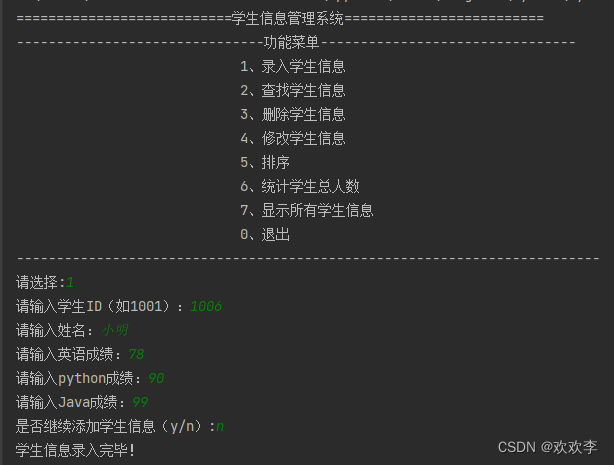2、查找学生信息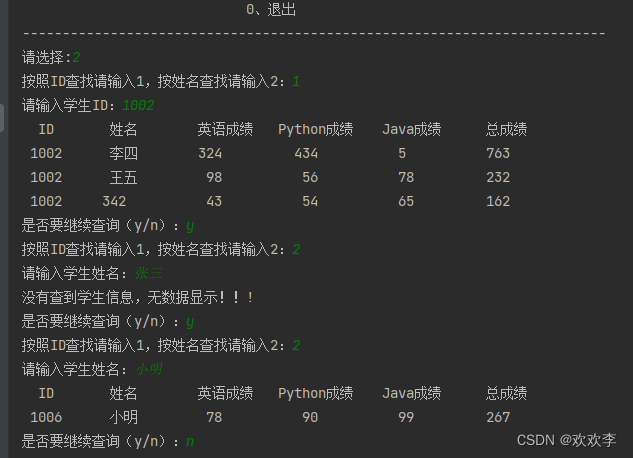3、删除学生信息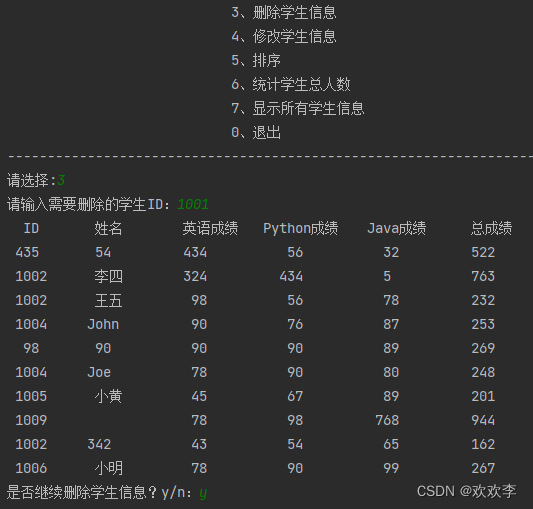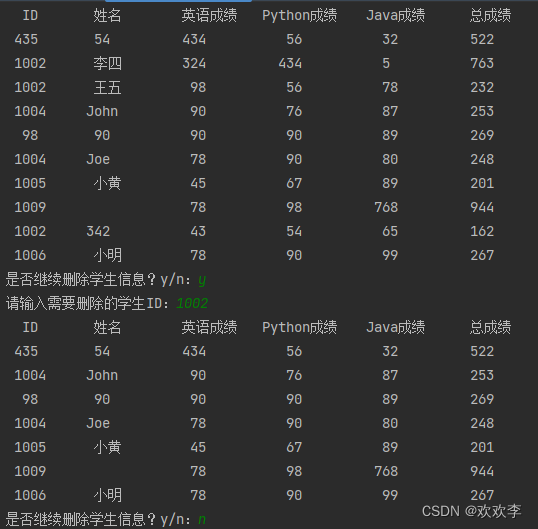4、修改学生信息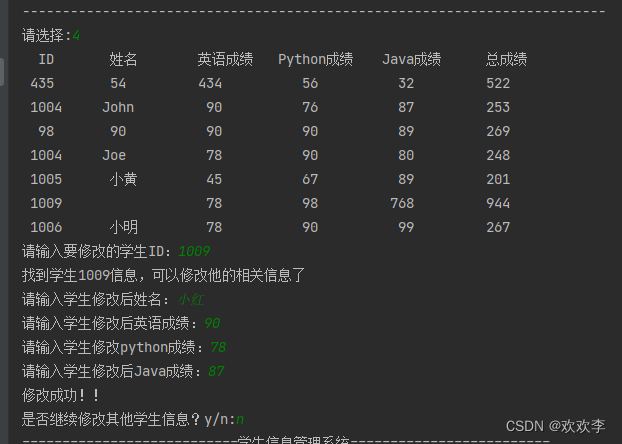5、查询学生信息，1009是刚刚修改的学生信息（没做相同的学生ID修改功能）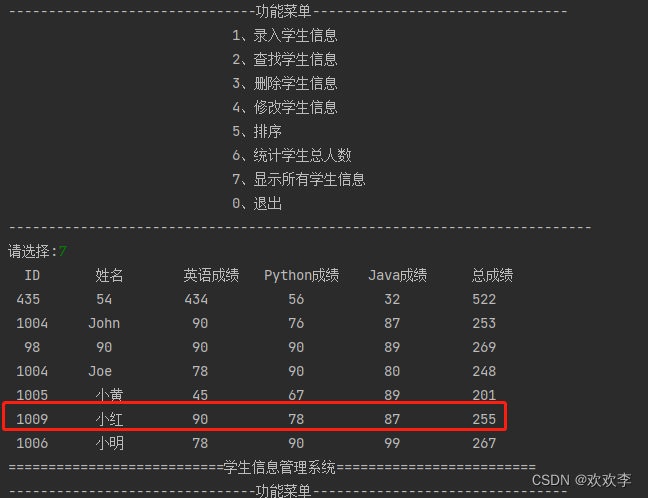6、排序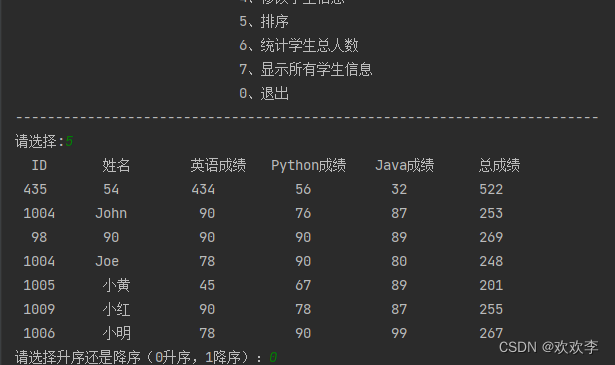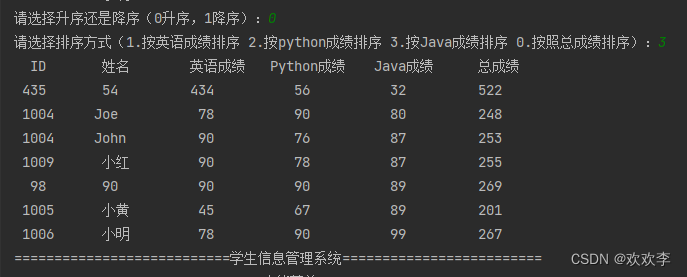7、统计学生总人数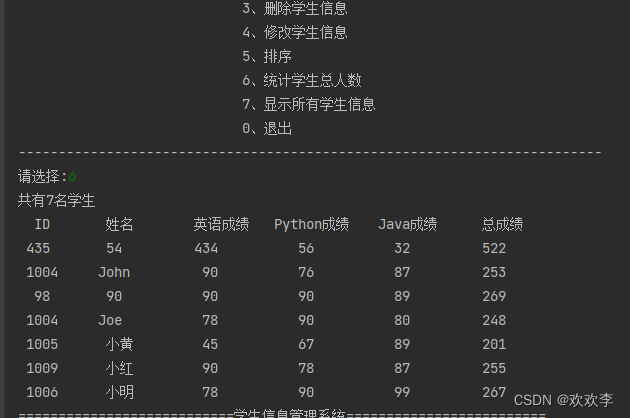8、退出功能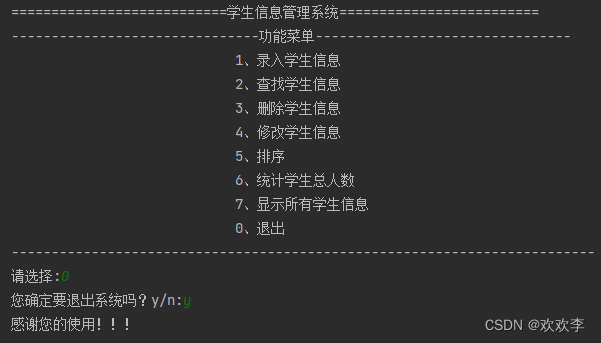### “python实现一个学生管理系统”的评论:

##### 关于作者##### overfit同步小助手